# TensorFlow 2.0 迁移学习实践指南## 架构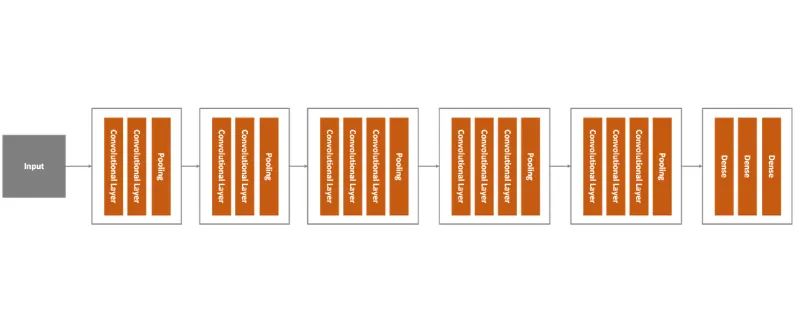VGG16 架构

GoogLeNet 也被称为 Inception，这是因为它使用了两个概念：1x1 卷积和 Inception 模块。第一个概念中，1x1 卷积用于降维的模块。通过降维，计算量也会减少，这也就意味着网络的深度和宽度可以增加了。GooLeNet 使用了 Inception 模块，每个卷积层的大小都不相同。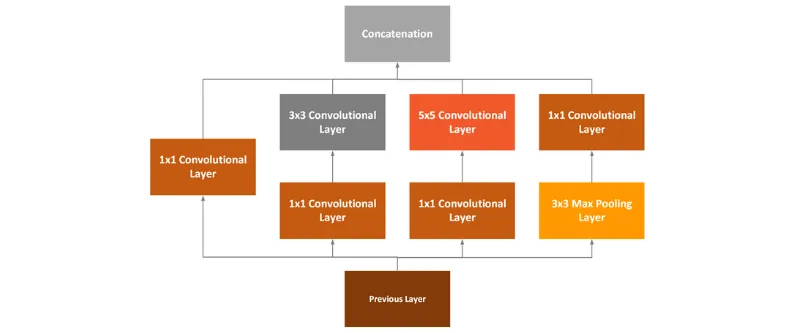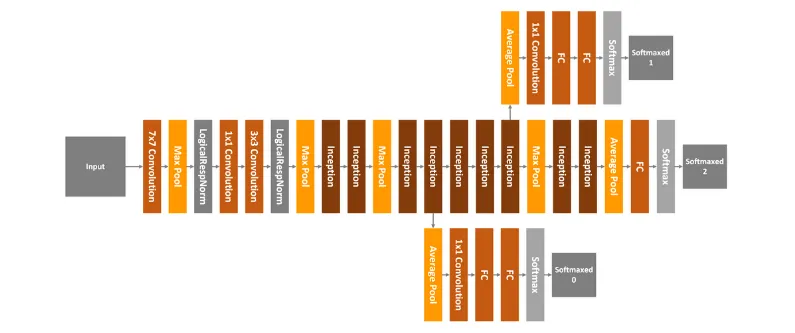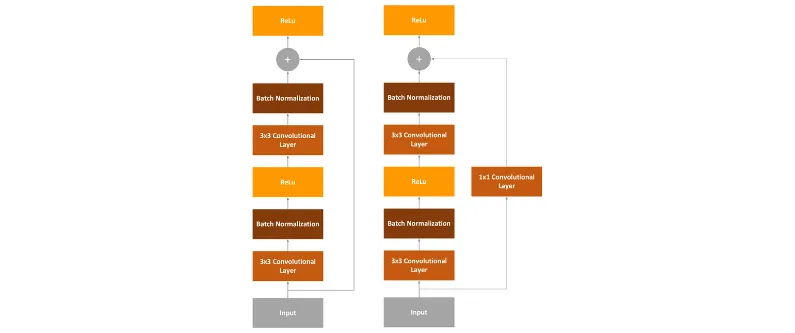## 数据集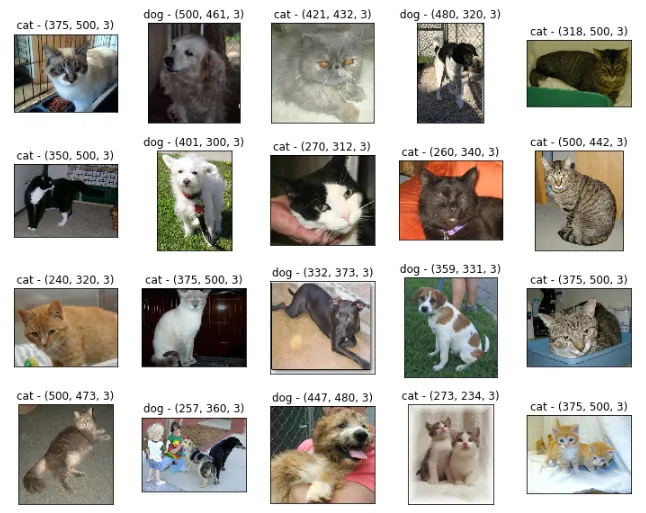pip install tensorflow-dataset

## 实现

import numpy as npimport matplotlib.pyplot as plt import tensorflow as tfimport tensorflow_datasets as tfds IMG_SIZE = 160BATCH_SIZE = 32SHUFFLE_SIZE = 1000IMG_SHAPE = (IMG_SIZE, IMG_SIZE, 3)

## 数据载入器

class DataLoader(object):    def __init__(self, image_size, batch_size):                self.image_size = image_size        self.batch_size = batch_size                # 80% train data, 10% validation data, 10% test data        split_weights = (8, 1, 1)        splits = tfds.Split.TRAIN.subsplit(weighted=split_weights)                (self.train_data_raw, self.validation_data_raw, self.test_data_raw), self.metadata = tfds.load(            'cats_vs_dogs', split=list(splits),            with_info=True, as_supervised=True)                # Get the number of train examples        self.num_train_examples = self.metadata.splits['train'].num_examples*80/100        self.get_label_name = self.metadata.features['label'].int2str                # Pre-process data        self._prepare_data()        self._prepare_batches()            # Resize all images to image_size x image_size    def _prepare_data(self):        self.train_data = self.train_data_raw.map(self._resize_sample)        self.validation_data = self.validation_data_raw.map(self._resize_sample)        self.test_data = self.test_data_raw.map(self._resize_sample)        # Resize one image to image_size x image_size    def _resize_sample(self, image, label):        image = tf.cast(image, tf.float32)        image = (image/127.5) - 1        image = tf.image.resize(image, (self.image_size, self.image_size))        return image, label        def _prepare_batches(self):        self.train_batches = self.train_data.shuffle(1000).batch(self.batch_size)        self.validation_batches = self.validation_data.batch(self.batch_size)        self.test_batches = self.test_data.batch(self.batch_size)       # Get defined number of  not processed images    def get_random_raw_images(self, num_of_images):        random_train_raw_data = self.train_data_raw.shuffle(1000)        return random_train_raw_data.take(num_of_images)

• _prepare_data：内部方法，用于缩放和归一化数据集里的图像。构造函数需要用到该函数。
• _resize_sample：内部方法，用于缩放单张图像。
• _prepare_batches：内部方法，用于将图像打包创建为 batches。创建 train_batches、validation_batches 和 test_batches，分别用于训练、评估过程。
• get_random_raw_images：这个方法用于从原始的、没有经过处理的数据中随机获取固定数量的图像。

def __init__(self, image_size, batch_size):     self.image_size = image_size    self.batch_size = batch_size     # 80% train data, 10% validation data, 10% test data    split_weights = (8, 1, 1)    splits = tfds.Split.TRAIN.subsplit(weighted=split_weights)     (self.train_data_raw, self.validation_data_raw, self.test_data_raw), self.metadata = tfds.load(        'cats_vs_dogs', split=list(splits),        with_info=True, as_supervised=True)     # Get the number of train examples    self.num_train_examples = self.metadata.splits['train'].num_examples*80/100    self.get_label_name = self.metadata.features['label'].int2str     # Pre-process data    self._prepare_data()    self._prepare_batches()

data_loader = DataLoader(IMG_SIZE, BATCH_SIZE) plt.figure(figsize=(10, 8))i = 0for img, label in data_loader.get_random_raw_images(20):    plt.subplot(4, 5, i+1)    plt.imshow(img)    plt.title("{} - {}".format(data_loader.get_label_name(label), img.shape))    plt.xticks([])    plt.yticks([])    i += 1plt.tight_layout()plt.show()## 基础模型 & Wrapper

vgg16_base = tf.keras.applications.VGG16(input_shape=IMG_SHAPE, include_top=False, weights='imagenet')googlenet_base = tf.keras.applications.InceptionV3(input_shape=IMG_SHAPE, include_top=False, weights='imagenet')resnet_base = tf.keras.applications.ResNet101V2(input_shape=IMG_SHAPE, include_top=False, weights='imagenet')

class Wrapper(tf.keras.Model):    def __init__(self, base_model):        super(Wrapper, self).__init__()                self.base_model = base_model        self.average_pooling_layer = tf.keras.layers.GlobalAveragePooling2D()        self.output_layer = tf.keras.layers.Dense(1)            def call(self, inputs):        x = self.base_model(inputs)        x = self.average_pooling_layer(x)        output = self.output_layer(x)        return output

base_learning_rate = 0.0001 vgg16_base.trainable = Falsevgg16 = Wrapper(vgg16_base)vgg16.compile(optimizer=tf.keras.optimizers.RMSprop(lr=base_learning_rate),              loss='binary_crossentropy',              metrics=['accuracy']) googlenet_base.trainable = Falsegooglenet = Wrapper(googlenet_base)googlenet.compile(optimizer=tf.keras.optimizers.RMSprop(lr=base_learning_rate),              loss='binary_crossentropy',              metrics=['accuracy']) resnet_base.trainable = Falseresnet = Wrapper(resnet_base)resnet.compile(optimizer=tf.keras.optimizers.RMSprop(lr=base_learning_rate),              loss='binary_crossentropy',              metrics=['accuracy'])

## 训练

steps_per_epoch = round(data_loader.num_train_examples)//BATCH_SIZEvalidation_steps = 20 loss1, accuracy1 = vgg16.evaluate(data_loader.validation_batches, steps = 20)loss2, accuracy2 = googlenet.evaluate(data_loader.validation_batches, steps = 20)loss3, accuracy3 = resnet.evaluate(data_loader.validation_batches, steps = 20) print("--------VGG16---------")print("Initial loss: {:.2f}".format(loss1))print("Initial accuracy: {:.2f}".format(accuracy1))print("---------------------------") print("--------GoogLeNet---------")print("Initial loss: {:.2f}".format(loss2))print("Initial accuracy: {:.2f}".format(accuracy2))print("---------------------------") print("--------ResNet---------")print("Initial loss: {:.2f}".format(loss3))print("Initial accuracy: {:.2f}".format(accuracy3))print("---------------------------")

———VGG16———Initial loss: 5.30Initial accuracy: 0.51—————————- ——GoogLeNet—–Initial loss: 7.21Initial accuracy: 0.51—————————- ——–ResNet———Initial loss: 6.01Initial accuracy: 0.51—————————-

history = vgg16.fit(data_loader.train_batches,                    epochs=10,                    validation_data=data_loader.validation_batches)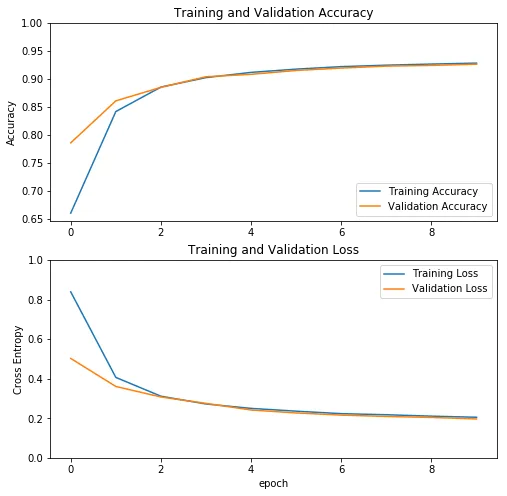VGG16 的训练过程历史数据

history = googlenet.fit(data_loader.train_batches,                    epochs=10,                    validation_data=data_loader.validation_batches)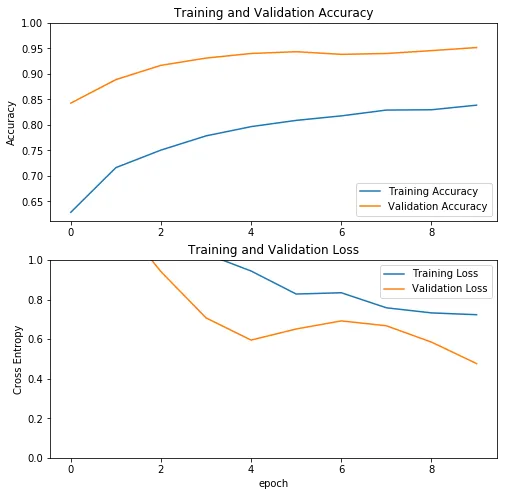history = resnet.fit(data_loader.train_batches,                    epochs=10,                    validation_data=data_loader.validation_batches)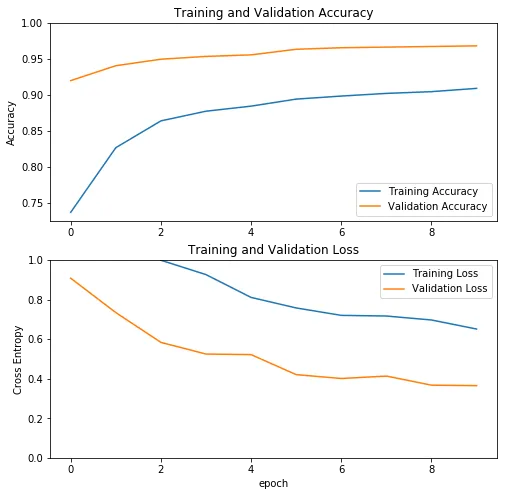ResNet 的训练过程历史数据

## 评估

loss1, accuracy1 = vgg16.evaluate(data_loader.test_batches, steps = 20)loss2, accuracy2 = googlenet.evaluate(data_loader.test_batches, steps = 20)loss3, accuracy3 = resnet.evaluate(data_loader.test_batches, steps = 20) print("--------VGG16---------")print("Loss: {:.2f}".format(loss1))print("Accuracy: {:.2f}".format(accuracy1))print("---------------------------") print("--------GoogLeNet---------")print("Loss: {:.2f}".format(loss2))print("Accuracy: {:.2f}".format(accuracy2))print("---------------------------") print("--------ResNet---------")print("Loss: {:.2f}".format(loss3))print("Accuracy: {:.2f}".format(accuracy3))print("---------------------------")

——–VGG16———Loss: 0.25Accuracy: 0.93————————— ——–GoogLeNet———Loss: 0.54Accuracy: 0.95———————————–ResNet———Loss: 0.40Accuracy: 0.97—————————

## 结论

https://rubikscode.net/2019/11/11/transfer-learning-with-tensorflow-2/